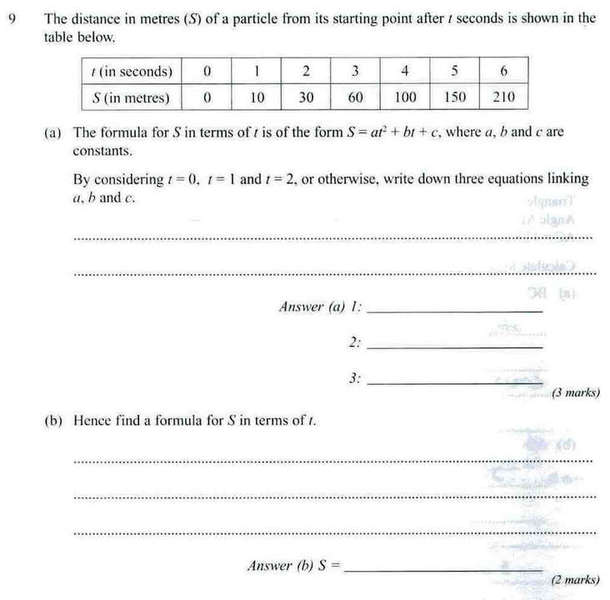# A equation that im stuck on

thomas49th

## Homework Statement## Homework Equations

As in the image above

## The Attempt at a Solution

I had a go but i rubbed it off the sheet.

Can someone take me through this step by step on how to solve it

Thanks

## Answers and Replies

Homework Helper
What is your question? You are told exactly what to do!

You are told that the equation must be of the form
S= at2+ bt+ c.

You are given t and S values for 6 different values of t.
Putting the given x and t values into the equation above for 3 different values of t (t= 0, 2, 3, as they suggest, will do but the choice is yours) will give you 3 equations to solve for a, b, and c. (Taking t= 0 will get an especially easy equation!)

thomas49th
so

t=0: 0 = 0 + 0 + 0 (c will have to be 0 if a and b are)
t=1: 10 =a+b+c
t=2: 30 =4a+2b+c
is that right?

Last edited:
jing
so

t=0: 0 = 0 + 0 + 0 (c will have to be 0 if a and b are)

but where does it say a and b are 0?

t=0: 0 = a.0 + b.0 +c and hence c=0

the other equations are correct

thomas49th
if t = 0
then a x t = 0
b x t = 0
so c must be 0 if the equation equals 0

jing
Correct. Now use that you know c=0 in the other two equations

thomas49th
Huh? I thought the other 2 equations were right?

t=0: 0 = 0a + 0b + 0
t=1: 10 =a+b+c
t=2: 30 =4a+2b+c

cant I right them as that?

jing
Yes they are correct but you now know that c = 0

so a+b+c=a+b

and

4a+2b+c=4a + 2b